The Falling Pedestrian (more correctly The Toppling Pedestrian)

by Eddie Baart

This problem was set by Duane Meyers. He wrote as follows.

"I would like your thoughts on a possible homicide involving a pedestrian who fell from a ledge at a parking ramp, down to a sidewalk below.

Facts

The top of the ledge is a vertical distance of 44.0 feet from the ground. The ped was found approximately 12 feet horizontally from the edge of the ledge. His CM is believed to be approximately 35 inches from the bottom of his feet.

Questions

What would the ped's horizontal velocity be if you assumed that the ped's body were rigid from the feet to the CM and he tipped out away from the ledge while standing on it ?

What would be the probable horizontal distance traveled from the ledge to the point of impact ?"The Solution

Suppose the pedestrian is of height l (l/2 is distance from center of gravity to his feet). We describe the motion of the center of gravity G in terms of the coordinates 2 and r as shown in the diagram. The x coordinate of G is l/2 cos q so its x component of velocity (by differentiation) isand its x component of acceleration is...............(1)

The two terms in the x acceleration can be recognized as the horizontal components of the tangential accelerationand the radial (centripetal) acceleration.

There is a radial acceleration as in uniform circular motion because, although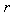is not changing in magnitude, its direction is changing.

The feet of the pedestrian will lose contact with the ledge when the horizontal component of the force exerted on them by the ledge (H in the diagram) becomes zero i.e. when the x component of the acceleration shown above becomes zero.

In order to find the angle at which this occurs we need to find expressions forand. To do this we use Newton's Second Law (for rotation) and Conservation of Energy.

Newton's Second Law

For rotational motion this law states that the moment of inertia times the angular acceleration equals the torque ( by analogy with ma = F for linear motion). The moment of inertia of the pedestrian for an axis through his feet is 1/3 l2 . So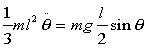and hence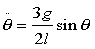....................................(2)

Conservation of Energy

The rotational kinetic energy when the pedestrian is at an anglemust equal the gravitational potential energy it has lost as his center of mass (CM) descended from its previous height of l/2 above his feet to the new height l/2 cos q. Thus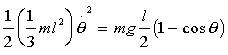and so........................(3)

The angle of launch

We now use the condition for the horizontal force to be zero i.e. that the x acceleration of equation (1) is zero.

If we substitute equations (2) and (3) into equation (1) and put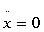, we getand henceIt is noteworthy that this result is independent of l and so it applies to the pedestrian as well as to a pencil toppling off a table.

The components of launch velocity

We can get the horizontal component of his velocity at the instant his feet leave the ledge by substituting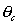into the equation (3) to findand then using the first equation on page 1.

This gives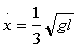...........................(4)and we can get a similar expression for the vertical speed when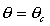.........(5)

Duane's Problem

We can apply equations (4) and (5) to Duane's problem with l = 2 x 0.889 m (2 x 35 inches).

The height of the CM at launch is l/2 cos q above his feet = 0.593 m and vertical velocity is 1.557 m/s.

So it falls 0.593m + 13.41 m (44 ft) = 14.00 m and this takes 1.54 seconds.

Since the horizontal launch velocity is 1.391 m/s he travels a horizontal distance of 2.14 metres (7.03 ft).

If we compare this with Duane's distance of 12 feet, then it is obvious that this was not a simple topple with the assumptions I have used. Whether foul play occurred, I leave you to judge.

************************************************